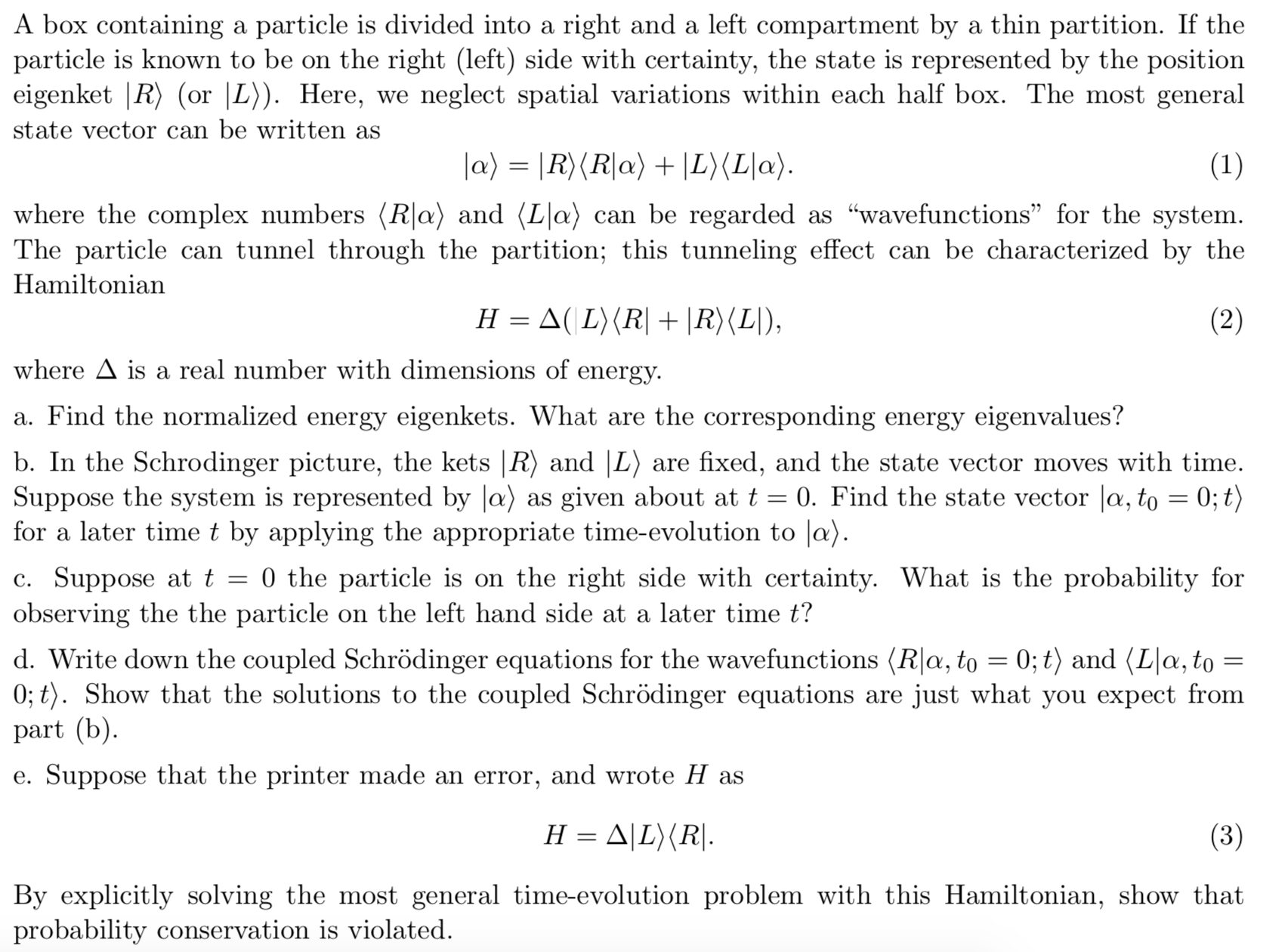# A box containing a particle is divided into a right and a left compartment by a thin partition. If theparticle is known to be on the right (left) side with certainty, the state is represented by the positioneigenket |R) (or |L)). Here, we neglect spatial variations within each half box. The most generalstate vector can be written asla) |R)(Ra) |LX(L\a).(1)where the complex numbers (R\a) and (Lla) can be regarded as "wavefunctions" for the systemThe particle can tunnel through the partition; this tunneling effect can be characterized by theHamiltonianA(L){R] + |R){L|),НЕ(2)where Ais a real number with dimensions of energya. Find the normalized energy eigenkets. What are the corresponding energy eigenvalues?b. In the Schrodinger picture, the kets |R) and |L) are fixed, and the state vector moves with time.Suppose the system is represented by a) as given about at t = 0. Find the state vector |a, to = 0; t)for a later time t by applying the appropriate time-evolution to la)c. Suppose at tobserving the the particle on the left hand side at a later time t?= 0 the particle is on the right side with certainty. What is the probability ford. Write down the coupled Schrödinger equations for the wavefunctions (R|a, to0; t). Show that the solutions to the coupled Schrödinger equations are just what you expect frompart (b)0; t) and (Lla, toe. Suppose that the printer made an error, and wrote H asH A|L)(R\.(3)By explicitly solving the most general time-evolution problem with this Hamiltonian, show thatprobability conservation is violated

Question
157 views

It's a quantum mechanics problem.help_outlineImage TranscriptioncloseA box containing a particle is divided into a right and a left compartment by a thin partition. If the particle is known to be on the right (left) side with certainty, the state is represented by the position eigenket |R) (or |L)). Here, we neglect spatial variations within each half box. The most general state vector can be written as la) |R)(Ra) |LX(L\a). (1) where the complex numbers (R\a) and (Lla) can be regarded as "wavefunctions" for the system The particle can tunnel through the partition; this tunneling effect can be characterized by the Hamiltonian A(L){R] + |R){L|), НЕ (2) where Ais a real number with dimensions of energy a. Find the normalized energy eigenkets. What are the corresponding energy eigenvalues? b. In the Schrodinger picture, the kets |R) and |L) are fixed, and the state vector moves with time. Suppose the system is represented by a) as given about at t = 0. Find the state vector |a, to = 0; t) for a later time t by applying the appropriate time-evolution to la) c. Suppose at t observing the the particle on the left hand side at a later time t? = 0 the particle is on the right side with certainty. What is the probability for d. Write down the coupled Schrödinger equations for the wavefunctions (R|a, to 0; t). Show that the solutions to the coupled Schrödinger equations are just what you expect from part (b) 0; t) and (Lla, to e. Suppose that the printer made an error, and wrote H as H A|L)(R\. (3) By explicitly solving the most general time-evolution problem with this Hamiltonian, show that probability conservation is violated fullscreen
check_circle

star
star
star
star
star
1 Rating
Step 1

Since we only answer up to 3 sub-parts, we’ll answer the first 3. Please resubmit the question and specify the other subparts (up to 3) you’d like answered.

Part(a)

Let the normalized eigenstates be:

Step 2

Due to linear independence of |L> and |R>:

Step 3

These define the two-level ...

### Want to see the full answer?

See Solution

#### Want to see this answer and more?

Solutions are written by subject experts who are available 24/7. Questions are typically answered within 1 hour.*

See Solution
*Response times may vary by subject and question.
Tagged in